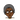BlogWork# Erlang Pattern Matching

June 15, 2021

If you come from a conventional programming language background, the way Erlang handles assignment is expected to look wonky, but it is not.

There is nothing like an assignment in Erlang programming language; there is a different approach to accessing values in memory, which is the pattern-matching operations.

With Java, PHP, Python, C, C++, and likes, the `=` symbol implies take the values from the right, and store it into the memory, then give me the reference of the location in memory and store it in the expression at the left.

Such that `const name = "Aleem Isiaka"` is correct in JavaScript, similar constructs exist in other languages using this concept.

In Erlang, `X = 20` reads very differently.

The Erlang runtime Beam understands it as `Evaluate the expressions at the right, match it with the value of the expressions at the left`.

Clarifying this further, the runtime first evaluates 20, which is a valid Erlang term (integer), then goes to the left to check if the X has a value already; in our case, it does not, meaning the X variable is unbound, an unbound variable will match any value at the right of the match operator (=).

This is what happens when we do `X = 20`. Did you get it?

The above example might seem off, let’s take this a little bit further. Consider the below Erlang snippet:

```.css-1baulvz{display:inline-block;}X = 20.Y = 30.Z = 30.
X = Y. %% -> Fails, 20 is not equals 30Y = X. %% -> Fails, 30 is not equals 20
Y = 30. %% -> Works, 30(Y) = 3030 = Y. %% -> Works, 30 = Y(30)Z = Y. %% Works, 30 = 30Y = Z. %% Works 30 = 30
%% Works?someFuncThatReturns30 = func() -> 30.Y = someFuncThatReturns30(). %% -> Sure, it does, as long as it returns a value that Y has been bounded to before now or Y is an unbound variable```

First, we have X matched(bounded, nothing like initialization in Erlang) to 20, with Y and Z bounded to 30. What we should note here is that:

Matching bounded variables with different values would throw an exception, like where `X = Y` or `Y = X` fails the match operation since `20` is not equal `30`.

• Matching bounded variables with hardcoded Erlang terms like atoms, binaries integers would match as long as both sides have the same value. This is why an initialized Y is equal to an equivalent of its bounded value 20
• Matching variables bounded differently would succeed as long as both variables contain the same value.
• The return value of a function (func in Erlang) would be successful in matching a variable that holds the same value as the returned value or an unbound variable. For example, an already bounded `Y` would always check the return value of `someFuncThatReturns30` as they are both equal.

Yes, that is all to Erlang pattern matching, but you might be wondering, how can I build a robust system if I can’t match a variable to another value that it is bounded to? Or do I can run out of names if I keep needing variables. You are not alone; I was here too!

The simple answer to this question is that: Erlang is a modular programming language and expects that you write your programs in modules. And modules are a way to group functions in a program.

Thus, in Erlang, variables have lexical scoping and only live in the context/function/process they are declared.

This does not make an Erlang program only scalable, and it also makes it more predictable as you don’t expect a variable to have two different values in the same context.

Variable names can be duplicated in different contexts:

```someFunc = (X) ->    x -> 20;    y -> 30.
anotherFun = (X) ->    all -> 100;    half -> 50.```

`someFunc` takes in one argument, which is pattern matched against `x` or `y`, both of which are atoms to return the requested value. While `anotherFunc` takes in one argument and pattern matching against `all` or `half` to return the requested value.

We can notice that both have `X` as the name of their argument; this is a valid Erlang module code that would be successful if it runs.

The operation’s success illustrates that we have to be careful of the context we are in not to bind a variable to an already bounded variable and pattern match correctly.

With these, I believe you have the basics of Erlang Pattern Matching.

Pattern matching is an excellent tool in writing programs for Erlang and other languages that depend on its VM.

Now that you have its basic understanding move up the ladder to know about functions.

Namaste!

Edit on githubTweetWith 💗 by Aleem Isiaka.
Software Engineer >>> Computer && Machines | Learner && Writer